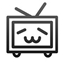﻿ Tensorflow BatchNormalization详解：1_原理及细节

# Batch Normalization: 原理及细节[mu_B leftarrow frac{1}{m}sum_{i=1}^m x_i ]

[sigma_{B}^{2} leftarrow frac{1}{m}sum_{i=1}^m (x_i - mu_B)^2 ]

[hat{x_i} leftarrow frac{x_i - mu_B}{sqrt{sigma_{B}^{2} + epsilon}} ]

[y_i leftarrow gamma hat{x_i} + eta ]